# 论文内容

1. 查找孔洞的边缘，计算边缘像素点的填充优先级
2. 挑选出优先级最高的点，根据像素点得到像素块
3. 在剩余部分查找和像素块最相似的像素块
4. 用最佳像素块填充对应的部分填充对应的孔洞部分，查看填好了没有，没有跳到1

## 优先级的计算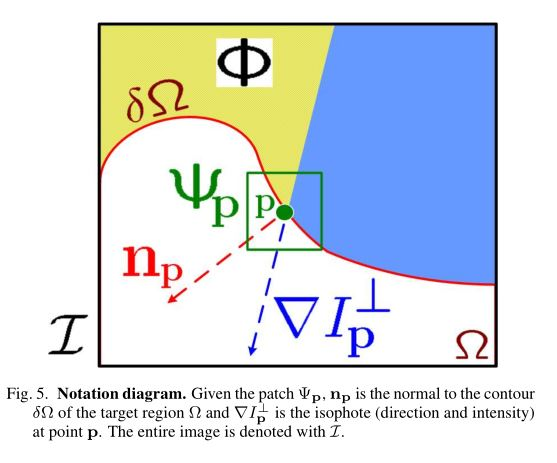P ( p ) = C ( p ) D ( p ) P(p)=C(p)D(p)

C ( p ) = ∑ q ∈ Ψ p ∩ ( I − Φ ) C ( q ) ∣ Ψ p ∣ C(p)=\frac{\sum_{q\in\Psi_p\cap(I-\Phi)C(q)}}{|\Psi_p|}

D ( p ) = ∣ ▽ I 1 p ∙ n p ∣ α D(p)=\frac{|\bigtriangledown I_{\frac{1}{p}}\bullet n_p|}{\alpha}

# 实现中遇到的问题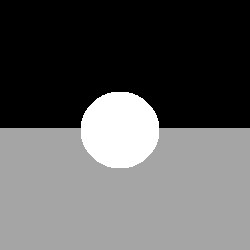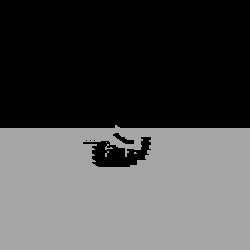d i f f = S Q D I F F − ∣ c o s ( θ ) ∣ + ∣ ∣ p ∣ − ∣ q ∣ ∣ diff=SQDIFF-|cos(\theta)|+||p|-|q||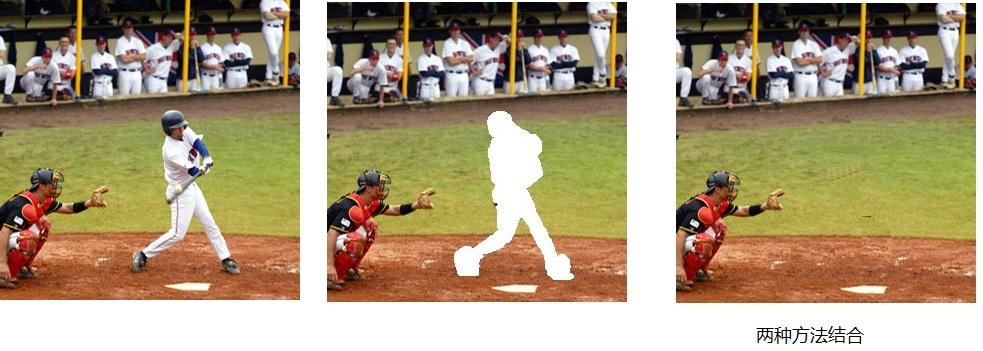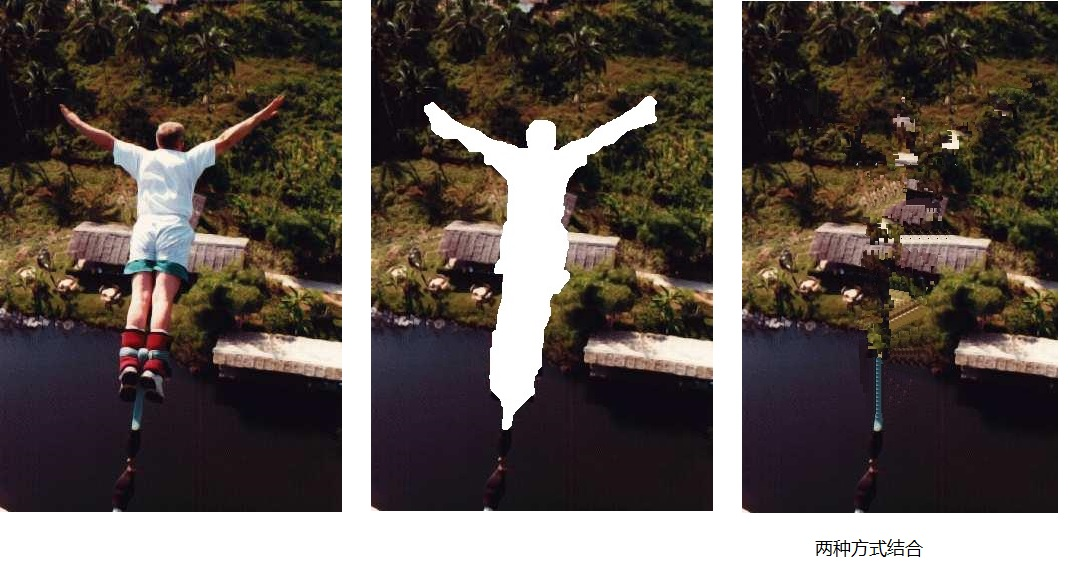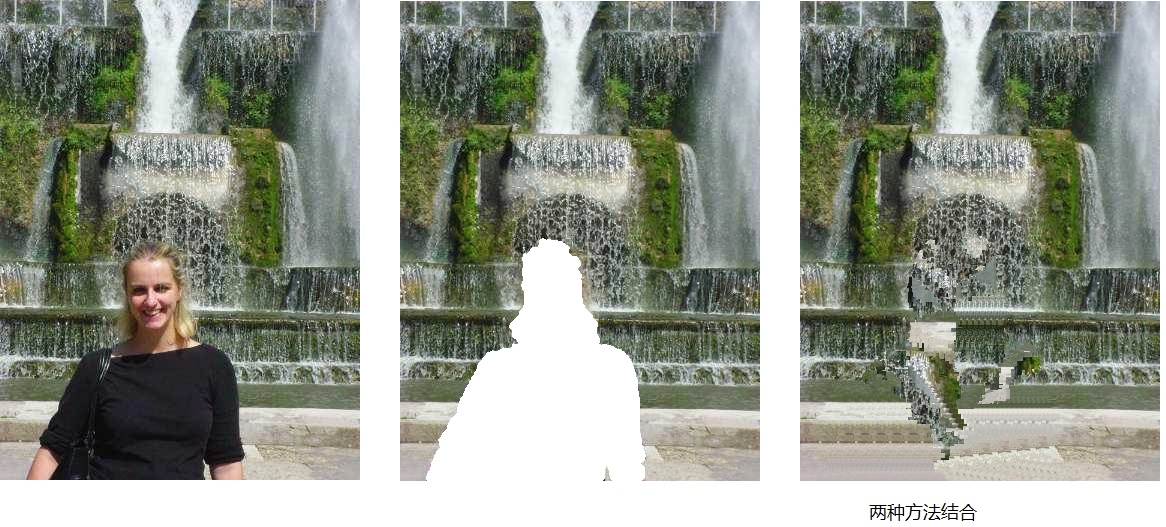# 总结

11-301万+01-289910
08-164227
02-285万+
11-19166
09-282320
06-231355
06-221958
12-163421